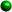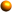Homework Tips for the WeekAdding & Subtracting Algebraic FractionsLike Denominators...keep the denominator and combine like terms in the numerator. Remember to put your answer in simplest form. Be careful with subtraction. Make sure your signs are correct, a minus in front of a parenthesis changes the sign of every term in the parenthesis.Unlike denominators... Get the common denominator and multiply by each term.To get the algebraic common denominator...each factor has to be represented and to the highest power that exists in any one term. If possible, factor first before getting your common denominator.Always look at your answer and ask yourself if there ios anything else to do, maybe factor or cancel. Solving Equations Involving Algebraic FractionsWe now have an equation, whatever we do to one side of the equation we can do to the other without upsetting the balance. We can multiply each side of the equation by the same thing. If we use what would be the common denominator, we can get rid of the fractions and have a nice little equation that is easy to solve.Decide what would be a common denominator and use that to multiply by each term in the equation. All denominators should cancel out to 1 and you should get a nice easy equation to solve without fractions.